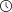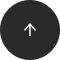- 9 minutes read

# What is the Difference Between COUNT(*), COUNT(1), COUNT(column name), and COUNT(DISTINCT column name)?

Have you noticed there are different variations of the SQL COUNT() function? This article explains the various arguments and their uses.

As a SQL user, you’re probably quite familiar with the `COUNT()` function. Even though it’s relatively simple, it can be used in several different ways. Each way has a very different use. I imagine you’ve seen code that contains the function `COUNT(*)` or `COUNT(1)`. You’ve probably also seen some other uses of the `COUNT()` function, such as `COUNT(column name)` and `COUNT(DISTINCT column name)`, even if you haven’t used them.

You’re probably wondering what each variation of `COUNT()` does. Let’s find out!

Before moving on, I want to say that I will use the GROUP BY function in this article, but only in a very simple way. If you need to refresh your memory on GROUP BY, here’s an article that’ll lead you through GROUP BY’s syntax and general principles. Or you can learn the fundamentals of `GROUP BY` in our SQL Basics course.

## What Does the COUNT() Function do?

As you can imagine, the `COUNT()` function counts. But what does it count? The `COUNT()` function belongs to SQL’s aggregate functions. It counts the number of rows that satisfy the criteria defined in the parentheses. It does not return the rows themselves; it shows the number of rows that meet your criteria.

Speaking of aggregate functions, they are extremely useful in SQL reports. If you want to satisfy your curiosity, there’s plenty of aggregate functions and “grouping by” in our Creating Basic SQL Reports course.

Now, back to counting. There are (naturally) different things that can be counted. That’s why there are different variations of the `COUNT()` function. In this article, I’ll concentrate on four:

• `COUNT(*)`
• `COUNT(1)`
• `COUNT(column name)`
• `COUNT(DISTINCT column name)`

## COUNT(*) vs COUNT(1)

You may have seen various discussions about the differences between `COUNT(*)` and `COUNT(1)`. And maybe trying to find the answer confused you even more. So, is there any difference? The simple answer is no – there is no difference at all.

The `COUNT(*)` function counts the total rows in the table, including the `NULL` values. The semantics for `COUNT(1)` differ slightly; we’ll discuss them later. However, the results for `COUNT(*)` and `COUNT(1)` are identical.

Let’s test this claim using an example query. Suppose I have a table named `orders` that contains these columns:

• `order_id`: The ID of the order.
• `customer_id`: The ID of the customer who placed the order.
• `order_value`: The total value of the ordered items, in euros.
• `payment_date`: When the order was paid by the customer.

If I wanted to count the number of rows in the whole table, I’d use the `COUNT()` function in the following way:

```SELECT COUNT(*) AS number_of_rows
FROM orders;
```

As you see, I’ve used the `COUNT(*)` function. The result will appear in the new column `number_of_rows`:

number_of_rows
8

Ok, but what if I use `COUNT(1)` instead? Here it is:

```SELECT COUNT(1) AS number_of_rows
FROM orders;
```

The code is essentially the same. The only difference is that I’ve used `COUNT(1)` instead of `COUNT(*)`. And what about the result? It returns the same number of rows:

number_of_rows
8

There’s a popular misconception that “1” in `COUNT(1)` means “count the values in the first column and return the number of rows.” From that misconception follows a second: that `COUNT(1)` is faster because it will count only the first column, while `COUNT(*)` will use the whole table to get to the same result.

This is not true. The number in the parenthesis doesn’t mean the number of the column in the table. If you put any number in the parenthesis, I guarantee you the result will be the same. Want proof? Here it is:

```SELECT COUNT(-13) AS number_of_rows
FROM orders;
```

If the first misconception was true, the code above would mean I want to count the number of rows in the -13th column. There are only four columns in the table `orders`, so there’s no 13th column. For sure, there is no column -13, whatever that should mean. Do you want to see the result of the code? Don't be surprised:

number_of_rows
8

Once again, the result is the same. So what does the value in the parenthesis of `COUNT()` mean? It’s the value that the `COUNT()` function will assign to every row in the table. The function will then count how many times the asterisk (*) or (1) or (-13) has been assigned. Of course, it will be assigned a number of times that’s equal to the number of rows in the table. In other words, `COUNT(1)` assigns the value from the parentheses (number 1, in this case) to every row in the table, then the same function counts how many times the value in the parenthesis (1, in our case) has been assigned; naturally, this will always be equal to the number of rows in the table. The parentheses can contain any value; the only thing that won’t work will be leaving the parentheses empty.

Let’s try something silly. Instead of a number, put the following value in the parenthesis: 'it will always be 8 rows'. Here’s the code:

```SELECT COUNT('it will always be 8 rows') AS number_of_rows
FROM orders;
```

Run the code and – surprise, surprise – the result is really eight rows:

number_of_rows
8

Since it doesn’t matter which value you put in the parentheses, it follows that `COUNT(*)` and `COUNT(1)` are precisely the same. They are precisely the same because the value in the `COUNT()` parentheses serves only to tell the query what it will count.

If these statements are precisely the same, then there’s no difference in the performance. Don’t let the asterisk (*) make you think it has the same use as in `SELECT *` statement. No, `COUNT(*)` will not go through the whole table before returning the number of rows, making itself slower than `COUNT(1)`.

So, in the end, who wins in this dramatic `COUNT(*)` vs `COUNT(1)` battle? Nobody – it’s a draw; they’re exactly the same. However, I’d recommend using `COUNT(*)`, as it’s much more commonly seen. It’s also less confusing, naturally leading other SQL users to understand that the function will count all the numbers in the table, including the `NULL` values.

## COUNT(*) vs COUNT(column name)

How about this one, `COUNT(*)` vs `COUNT(column name)`. Is there any difference? There sure is!

As you’ve already learned, `COUNT(*)` will count all the rows in the table, including `NULL` values. On the other hand, `COUNT(column name)` will count all the rows in the specified column while excluding `NULL` values.

As you already know, there are eight rows in the table `orders`. Let’s see how many rows there will be when I use the column `order_id` for counting (imagining I want to see how many orders have been placed). We’ll get eight rows again, right? Let’s see:

```SELECT COUNT(order_id) AS number_of_orders
FROM orders;
```

Do we get the same result? Nope, there are seven orders, not eight.

number_of_orders
7

Is that a mistake? No, it’s not; there are really only seven orders with an `order_id`; one row has a `NULL` instead of a proper `order_id`. Below is the row that makes the difference:

order_idcustomer_idorder_priceorder_date
NULLCU0921327.85NULL

Always remember: `COUNT(column name)` will only count rows where the given column is NOT NULL.

Let’s do something interesting now and combine both `COUNT()` variations in one query. Suppose I want to see the customer’s ID with the total number of orders by that customer. I also want to show the total number of paid orders for that customer. (Paid orders don’t have a `NULL` value in the column `payment_date`.) Here’s how I’d do it:

```SELECT	customer_id,
COUNT(*) AS number_of_orders,
COUNT(payment_date) AS paid_number_of_orders
FROM orders
GROUP BY customer_id;
```

The query will first calculate the total number of orders using `COUNT(*)` – i.e. it will include `NULL` values. Then the part `COUNT (payment_date) AS paid_number_of_orders` will count the rows in the column `payment_date` that are `NOT NULL`. I want the results for each customer, so I’ve grouped the result by the column `customer_id`. Here’s what I get:

customer_idnumber_of_orderspaid_number_of_orders
CU01211
CU04911
CU05222
CU09210
CU10822
CU14911

You can see that the difference occurs for the customer CU092.

The principles of combining `GROUP BY` and `COUNT()` are outlined in this article about GROUP BY and SQL aggregate functions. If you want some more practice, here are five examples of GROUP BY.

`COUNT()` allows us to use expressions as well as column names as the argument. Do you know how to find the number of the orders above €1 000 using only the `COUNT()` function? Here’s how:

```SELECT COUNT(CASE WHEN order_price > 1000 THEN 1 END)
AS significant_orders
FROM orders;
```

Instead of putting conditions at the end of the query and filtering after the `COUNT()` function does its job, we can use the `CASE` statement. That’s what I’ve done in the above query. It’s used like an IF-THEN-ELSE statement. `CASE` is followed by the condition, which is defined by the statements WHEN and THEN. There can also be an ELSE statement, but it’s unnecessary in this case – I’m only interested in counting the number of values, not in the values themselves. Every `CASE` statement ends with the `END` statement.

The `COUNT()` statement above reads as follows:

1. Find all the values in the column `order_price` above 1 000.
2. Assign the value 1 (you can assign any value you want) to these values.
3. Assign NULL to rows with prices below 1 000.
4. Count the number of assigned 1s.
5. Show the result in the column `significant_orders`.

Here’s the result:

significant_orders
5

## COUNT(column name) vs COUNT (DISTINCT column_name)

You can probably imagine what the difference between those two `COUNT()` function versions is. `COUNT(column_name)` will include duplicate values when counting. In contrast, `COUNT (DISTINCT column_name)` will count only distinct (unique) rows in the defined column.

If you want to count the number of customers who’ve placed an order, maybe `COUNT (column_name)` will work. Let’s try this simple code:

```SELECT COUNT (customer_id) AS number_of_customers
FROM orders;
```

You’re familiar with this one; I’ve already used the `COUNT(column name)` function. This time it counts all rows in the column `customer_id`, with the result being shown in the column `number_of_customers`. Here’s the result:

number_of_customers
8

Let’s check the result by looking at the entire `orders` table:

order_idcustomer_idorder_priceorder_date
OR2020-01CU108154872020-01-08
OR2020-28CU149154872020-01-14
OR2020-12CU10812549.222020-01-09
OR2020-91CU012542.55NULL
NULLCU0921327.85NULL
OR2020-112CU049150002020-02-28
OR2020-213CU0521502020-03-12
OR2020-213CU0522002020-03-12

There are eight rows, but is this really the number of the customers? Notice that the customers CU108 and CU052 appear twice. If I want the real number of customers, then I need to count every customer only once. How can I do this? By using `COUNT(DISTINCT customer_id)`:

```SELECT COUNT(DISTINCT customer_id) AS number_of_customers
FROM orders;
```

This query will also count rows in the column `customer_id`, but it will count every customer only once. This is due to the keyword `DISTINCT`. Have a look at the result:

number_of_customers
6

This is the correct result; there are really only six unique customers.

## Do You Think You Can Count on COUNT()?

Now that you understand several common variations of the `COUNT()` function, you can create more complex calculations and reports. `COUNT()` is one of the most used aggregate functions, so it’s vital that you clearly understand the different `COUNT()` variations and their purposes. If some of the `COUNT()` function variations we discussed in this article weren’t clear, let me know in the comment section. I’ll gladly help you. And, for more practice using `COUNT()`, try our Creating Basic SQL Reports course.